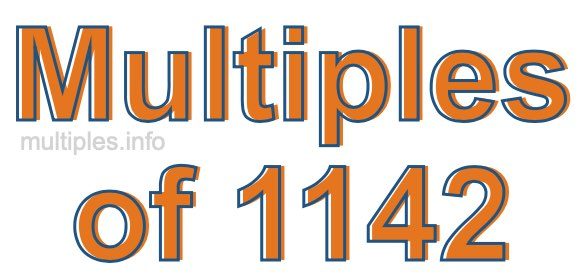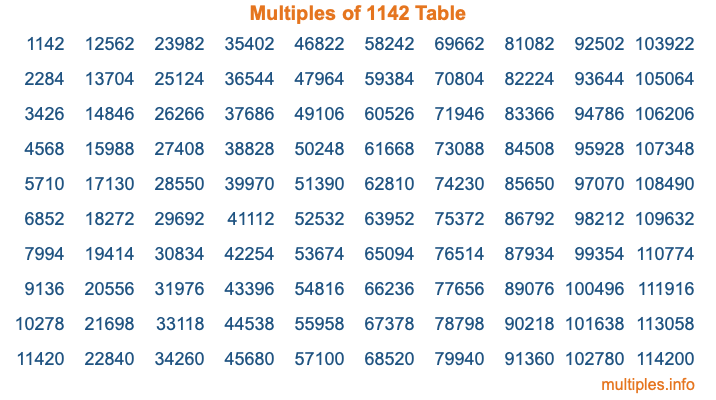Multiples of 1142Welcome to the Multiples of 1142 page. Here we will first teach you everything you will ever need to know about the multiples of 1142, and then give you a study guide summary of everything we taught you to make sure you remember it all. Use this page to look up facts and learn information about the multiples of 1142. This page will make you a multiples of one thousand one hundred forty-two expert!

Definition of Multiples of 1142
Multiples of 1142 are all the numbers that when divided by 1142 equal an integer. Each of the multiples of 1142 are called a multiple. A multiple of 1142 is created by multiplying 1142 by an integer.

Therefore, to create a list of multiples of 1142, you start with 1 multiplied by 1142, then 2 multiplied by 1142, then 3 multiplied by 1142, and so on for as long as you want. Thus, the list of the first five multiples of 1142 is 1142, 2284, 3426, 4568, and 5710. To see a larger list of multiples of 1142, see the printable image of Multiples of 1142 further down on this page. We also have a category where you can choose any nth multiple of 1142.

Multiples of 1142 Checker
The Multiples of 1142 Checker below checks to see if any number of your choice is a multiple of 1142. In other words, it checks to see if there is any number (integer) that when multiplied by 1142 will equal your number. To do that, we divide your number by 1142. If the the quotient is an integer, then your number is a multiple of 1142.

Is  a multiple of 1142?

Least Common Multiple of 1142 and ...
A Least Common Multiple (LCM) is the lowest multiple that two or more numbers have in common. This is also called the smallest common multiple or lowest common multiple and is useful to know when you are adding our subtracting fractions. Enter one or more numbers below (1142 is already entered) to find the LCM.

Check out our LCM Calculator if you need more details about the Least Common Multiple or if you need the LCM for different numbers for adding and subtraction fractions.

nth Multiple of 1142
As we stated above, 1142 is the first multiple of 1142, 2284 is the second multiple of 1142, 3426 is the third multiple of 1142, and so on. Enter a number below to find the nth multiple of 1142.

th multiple of 1142

Multiples of 1142 vs Factors of 1142
1142 is a multiple of 1142 and a factor of 1142, but that is where the similarities end. All postive multiples of 1142 are 1142 or greater than 1142. All positive factors of 1142 are 1142 or less than 1142.

Below is the beginning list of multiples of 1142 and the factors of 1142 so you can compare:

Multiples of 1142: 1142, 2284, 3426, 4568, 5710, etc.

Factors of 1142: 1, 2, 571, 1142

As you can see, the multiples of 1142 are all the numbers that you can divide by 1142 to get a whole number. The factors of 1142, on the other hand, are all the whole numbers that you can multiply by another whole number to get 1142.

It's also interesting to note that if a number (x) is a factor of 1142, then 1142 will also be a multiple of that number (x).

Multiples of 1142 vs Divisors of 1142
The divisors of 1142 are all the integers that 1142 can be divided by evenly. Below is a list of the divisors of 1142.

Divisors of 1142: 1, 2, 571, 1142

The interesting thing to note here is that if you take any multiple of 1142 and divide it by a divisor of 1142, you will see that the quotient is an integer.

Multiples of 1142 Table
Below is an image of the first 100 multiples of 1142 in a table. The table is in chronological order, column by column. The first column has the first ten multiples of 1142, the second column has the next ten multiples of 1142, and so on.The Multiples of 1142 Table is also referred to as the 1142 Times Table or Times Table of 1142. You are welcome to print out our table for your studies.

Negative Multiples of 1142
Although not often discussed or needed in math, it is worth mentioning that you can make a list of negative multiples of 1142 by multiplying 1142 by -1, then by -2, then by -3, and so on, to get the following list of negative multiples of 1142:

-1142, -2284, -3426, -4568, -5710, etc.

Multiples of 1142 Summary
Below is a summary of important Multiples of 1142 facts that we have discussed on this page. To retain the knowledge on this page, we recommend that you read through the summary and explain to yourself or a study partner why they hold true.

There are an infinite number of multiples of 1142.

A multiple of 1142 divided by 1142 will equal a whole number.

1142 divided by a factor of 1142 equals a divisor of 1142.

The nth multiple of 1142 is n times 1142.

The largest factor of 1142 is equal to the first positive multiple of 1142.

1142 is a multiple of every factor of 1142.

1142 is a multiple of 1142.

A multiple of 1142 divided by a divisor of 1142 equals an integer.

1142 divided by a divisor of 1142 equals a factor of 1142.

Any integer times 1142 will equal a multiple of 1142.

Multiples of a Number
Here you can get the multiples of another number, all with the same attention to detail as we did for multiples of 1142 on this page.

Multiples of
Multiples of 1143
Did you find our page about multiples of one thousand one hundred forty-two educational? Do you want more knowledge? Check out the multiples of the next number on our list!